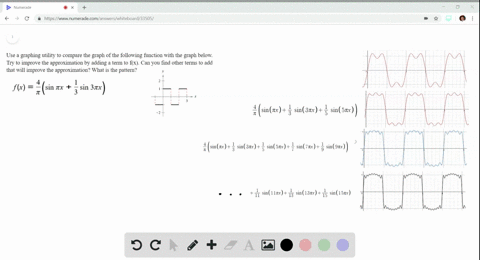🎉 The Study-to-Win Winning Ticket number has been announced! Go to your Tickets dashboard to see if you won! 🎉View Winning Ticket### Pattern Recognition Use a graphing utility to com…

03:37Oregon State University
Problem 75

# Sales The monthly sales $S$ (in thousands of units) of a seasonal product are modeled by$$S=58.3+32.5 \cos \frac{\pi t}{6}$$where $t$ is the time (in months), with $t=1$ corresponding to January. Use a graphing utility to graph the model for $S$ and determine the months when sales exceed $75,000$ units.

## Discussion

You must be signed in to discuss.

## Video Transcript

All right, let's using graphing utility for this problem. So here's my graphing calculator, and you can go into the y equals menu and type the function in there. So this is the function that represents the sales and thousands of units of the seasonal product. And I'm using X in my calculator in place of tea, and that would be the time in months. So X equals one is going to be January. So here's what the graph looks like. And for my window dimensions, I went from 0 to 12 for the months and then 0 to 140 for the units in thousands. Okay, so we're interested in knowing which months have sales greater than 100,000. So I'm going to go back to my Y, equals menu and go toe white, too, and put 100 there, which represents 100,000 and that's going to give us a horizontal line, and we want to know which months go above that line. So let's find those intersection points. Roughly. What we can do is move that cursor along, so this intersection point is at about 4.9, and then on the other side. This intersection point is at about 1.2. So then months 23 and four fallen between month to would be February 3 would be march and for would be April, so sales exceed 100,000 units in February, March and April.

#### You're viewing a similar answer. To request the exact answer, fill out the form below:

Our educator team will work on creating an answer for you in the next 6 hours.